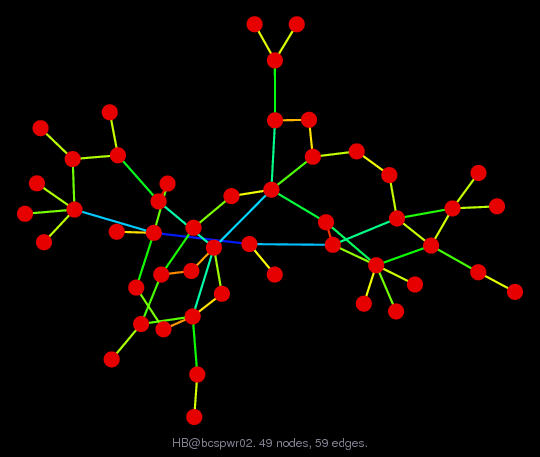Matrix: HB/bcspwr02

Description: SYMMETRIC STRUCTURE OF A SMALL TEST POWER SYSTEM(undirected graph drawing)• Matrix group: HB
• download as a MATLAB mat-file, file size: 782 bytes. Use UFget(14) or UFget('HB/bcspwr02') in MATLAB.

 Matrix properties number of rows 49 number of columns 49 nonzeros 167 structural full rank? yes structural rank 49 # of blocks from dmperm 1 # strongly connected comp. 1 explicit zero entries 0 nonzero pattern symmetry symmetric numeric value symmetry symmetric type binary structure symmetric Cholesky candidate? yes positive definite? no

 author B. Dembart, J. Lewis editor I. Duff, R. Grimes, J. Lewis date 1981 kind power network problem 2D/3D problem? no

 Ordering statistics: result nnz(chol(P*(A+A'+s*I)*P')) with AMD 129 Cholesky flop count 3.6e+02 nnz(L+U), no partial pivoting, with AMD 209 nnz(V) for QR, upper bound nnz(L) for LU, with COLAMD 149 nnz(R) for QR, upper bound nnz(U) for LU, with COLAMD 275

 SVD-based statistics: norm(A) 4.30857 min(svd(A)) 1.32075e-16 cond(A) 3.26221e+16 rank(A) 48 sprank(A)-rank(A) 1 null space dimension 1 full numerical rank? no singular value gap 5.75129e+14

 singular values (MAT file): click here SVD method used: s = svd (full (A)) ; status: ok